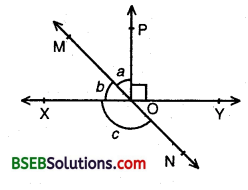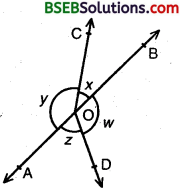# HSSlive: Plus One & Plus Two Notes & Solutions for Kerala State Board

## BSEB Class 9 Maths Chapter 6 Lines and Angles Ex 6.1 Textbook Solutions PDF: Download Bihar Board STD 9th Maths Chapter 6 Lines and Angles Ex 6.1 Book AnswersBSEB Class 9 Maths Chapter 6 Lines and Angles Ex 6.1 Textbook Solutions PDF: Download Bihar Board STD 9th Maths Chapter 6 Lines and Angles Ex 6.1 Book Answers

BSEB Class 9th Maths Chapter 6 Lines and Angles Ex 6.1 Textbooks Solutions and answers for students are now available in pdf format. Bihar Board Class 9th Maths Chapter 6 Lines and Angles Ex 6.1 Book answers and solutions are one of the most important study materials for any student. The Bihar Board Class 9th Maths Chapter 6 Lines and Angles Ex 6.1 books are published by the Bihar Board Publishers. These Bihar Board Class 9th Maths Chapter 6 Lines and Angles Ex 6.1 textbooks are prepared by a group of expert faculty members. Students can download these BSEB STD 9th Maths Chapter 6 Lines and Angles Ex 6.1 book solutions pdf online from this page.

## Bihar Board Class 9th Maths Chapter 6 Lines and Angles Ex 6.1 Books Solutions

 Board BSEB Materials Textbook Solutions/Guide Format DOC/PDF Class 9th Subject Maths Chapter 6 Lines and Angles Ex 6.1 Chapters All Provider Hsslive

## How to download Bihar Board Class 9th Maths Chapter 6 Lines and Angles Ex 6.1 Textbook Solutions Answers PDF Online?

2. Click on the Bihar Board Class 9th Maths Chapter 6 Lines and Angles Ex 6.1 Answers.
3. Look for your Bihar Board STD 9th Maths Chapter 6 Lines and Angles Ex 6.1 Textbooks PDF.
4. Now download or read the Bihar Board Class 9th Maths Chapter 6 Lines and Angles Ex 6.1 Textbook Solutions for PDF Free.

## BSEB Class 9th Maths Chapter 6 Lines and Angles Ex 6.1 Textbooks Solutions with Answer PDF Download

Find below the list of all BSEB Class 9th Maths Chapter 6 Lines and Angles Ex 6.1 Textbook Solutions for PDF’s for you to download and prepare for the upcoming exams:

## BSEB Bihar Board Class 9th Maths Solutions Chapter 6 Lines and Angles Ex 6.1

Question 1.
In figure, lines AB and CD intersect at O. If ∠AOC + ∠BOE = 70° and ∠BOD = 40°, find ∠BOE and reflex ∠COE.Solution:
Since OA and OB are opposite rays. Therefore, AB is a line.
Since ray OC stands on AB. Therefore,
∠AOC + ∠COB = 180° [Linear Pairs]
⇒ ∠AOC + ∠COE + ∠BOE = 180° [∵∠COB = ∠COE + ∠BOE]
⇒ (∠AOC + ∠BOE) + ∠COE = 180°
⇒ 70° + ∠COE = 180° [∵ ∠AOC + ∠BOE = 70° (Given)]
⇒ ∠COE = 180° – 70° = 110°
∴ Reflex ∠COE = 360° – 110° = 250°
Since OC and OD are opposite rays. Therefore, CD is a line.
Since ray OE stands on CD. Therefore,
∠COE + ∠EOD = 180° [Linear Pairs]
⇒ ∠COE) + ∠BOE + ∠BOD = 180°
⇒ 110° + ∠BOE + 40° = 180°
[∵ ∠COE = 110° (probed above), ∠BOD = 40° (Given)]
⇒ ∠BOE = 180° – 110° – 40° = 30°
Hence, ∠BOE = 30° and reflex ∠COE = 250°.

Question 2.
In figure, lines XY and MN intersect at O. If ∠POY = 90° and a : 6 = 2 : 3, find c.Solution:
Since a : b = 2 : 3 and a + b = ∠POX = ∠POY = 90° and sum- of ratios = 2 + 3 = 5
∴ a = 25 x 90° = 2 x 18° = 36°
and b = 35 x 90° = 3 x 18° = 54°
Since OM and ON are opposite rays. Therefore, MN is a line.
Since ray OX stands on MN. Therefore,
∠MOX + ∠XON = 180°’ [Linear Pairs]
⇒ c + b = 180° ⇒ c + 54° = 180°
⇒ c = 180° – 54° = 126°
Hence, c = 126°.

Question 3.
In figure, ∠PQR = ∠PRQ, then prove that ∠PQS = ∠PRT.Solution:
Since QS and QR are opposite rays. Therefore, SR is a line.
Since QP stands on the line SR.
∴ ∠PQS + ∠PQR = 180° [Linear Pair] … (1)
Again, RQ and RT are opposite rays. Therefore, QT is a line.
Since ER stands on the line QT.
∴ ∠PRQ + ∠PRT = 180° [Linear Pair] …(2)
From (1) and (2), we have
∠PQS + ∠PQR = ∠PRQ + ∠PRT [∵ Each side = 180°] … (3)
Also ∠PQR = ∠PRQ [Given] … (4)
Subtracting (4) from (3), we have ∠PQS = ∠PRT.

Question 4.
In figure, if x + y = w + z, then prove that AOB is a line.Solution:
Since the sum all angles round a point is equal to 360°Thus, ∠BOC and ∠COA, ∠BOD and ∠AOD form linear pairs. Consequently OA and OB are two opposite rays. Therefore, AOB is a straight line.

Question 5.
In figure, POQ is a line. Ray OR is perpendicular to line PQ. OS is another ray lying between rays OP and OR. Prove that ∠ROS = 12(∠QOS – ∠POS).Solution:
Since OR is perpendicular to the line PQ.
∴∠POR = ∠ROQ [∵ Each = 90°]
⇒ ∠POS + ∠ROS = ∠QOS – ∠ROS
⇒ 2∠ROS =∠QOS – ∠POS
⇒ ∠ROS = 12(∠QOS – ∠POS)

Question 6.
It is given that ∠XYZ = 64° and XY is produced to point P. Draw a figure from the given information. If ray YQ bisects ∠ZYP, find ∠XYQ and reflex ∠QYP.Solution:
Since XY is produced to point P. Therefore, XP is a straight line.
Since YZ stands on XP.
∴ ∠XYZ + ∠ZYP = 180° [Linear Pair]
⇒ 64° + ∠ZYP = 180° [∵ ∠XYZ = 64°]
⇒ ∠ZYP = 180° – 64° = 116°
Since ray YQ bisects ∠ZYP.
Therefore,∠QYP = ∠ZYQ = 1112 = 58°
Now, ∠XYQ = ∠XYZ + ∠ZYQ
⇒ ∠XYQ = 64° + 58° = 122°
and reflex ∠QYP = 360° – ∠QYP = 360° – 58° = 302°

## Bihar Board Class 9th Maths Chapter 6 Lines and Angles Ex 6.1 Textbooks for Exam Preparations

Bihar Board Class 9th Maths Chapter 6 Lines and Angles Ex 6.1 Textbook Solutions can be of great help in your Bihar Board Class 9th Maths Chapter 6 Lines and Angles Ex 6.1 exam preparation. The BSEB STD 9th Maths Chapter 6 Lines and Angles Ex 6.1 Textbooks study material, used with the English medium textbooks, can help you complete the entire Class 9th Maths Chapter 6 Lines and Angles Ex 6.1 Books State Board syllabus with maximum efficiency.

## FAQs Regarding Bihar Board Class 9th Maths Chapter 6 Lines and Angles Ex 6.1 Textbook Solutions

#### How to get BSEB Class 9th Maths Chapter 6 Lines and Angles Ex 6.1 Textbook Answers??

Students can download the Bihar Board Class 9 Maths Chapter 6 Lines and Angles Ex 6.1 Answers PDF from the links provided above.

#### Can we get a Bihar Board Book PDF for all Classes?

Yes you can get Bihar Board Text Book PDF for all classes using the links provided in the above article.

## Important Terms

Bihar Board Class 9th Maths Chapter 6 Lines and Angles Ex 6.1, BSEB Class 9th Maths Chapter 6 Lines and Angles Ex 6.1 Textbooks, Bihar Board Class 9th Maths Chapter 6 Lines and Angles Ex 6.1, Bihar Board Class 9th Maths Chapter 6 Lines and Angles Ex 6.1 Textbook solutions, BSEB Class 9th Maths Chapter 6 Lines and Angles Ex 6.1 Textbooks Solutions, Bihar Board STD 9th Maths Chapter 6 Lines and Angles Ex 6.1, BSEB STD 9th Maths Chapter 6 Lines and Angles Ex 6.1 Textbooks, Bihar Board STD 9th Maths Chapter 6 Lines and Angles Ex 6.1, Bihar Board STD 9th Maths Chapter 6 Lines and Angles Ex 6.1 Textbook solutions, BSEB STD 9th Maths Chapter 6 Lines and Angles Ex 6.1 Textbooks Solutions,
Share: